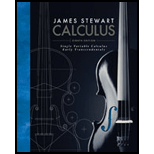# For any normal distribution, find the probability that the random variable lies within two standard deviations of the mean.### Single Variable Calculus: Early Tr...

8th Edition
James Stewart
Publisher: Cengage Learning
ISBN: 9781305270336### Single Variable Calculus: Early Tr...

8th Edition
James Stewart
Publisher: Cengage Learning
ISBN: 9781305270336

#### Solutions

Chapter
Section
Chapter 8.5, Problem 19E
Textbook Problem

## For any normal distribution, find the probability that the random variable lies within two standard deviations of the mean.

Expert Solution
To determine

To find: The probability that the random variable lies within two standard deviations of the mean.

### Explanation of Solution

Given information:

The function is normal distribution.

Calculation:

Show the probability density function for normal distribution as follows.

f(x)=1σ2πe(xμ)22σ2 (1)

Consider the standard means for normal distributions are μ2σ and μ+2σ .

Find the probability density that the random variable lies within two standard deviations of the mean P(μ2σXμ+2σ) as shown below.

P(μ2σXμ+2σ)=μ2σμ+2σ1σ2πe(xμ)22σ2dx (2)

Consider t=xμσ (3)

Differentiate both sides of the Equation.

dt=1σdx

Calculate the lower limit of t using Equation (3).

Substitute μ2σ for x in Equation (3)

### Want to see the full answer?

Check out a sample textbook solution.See solution

### Want to see this answer and more?

Bartleby provides explanations to thousands of textbook problems written by our experts, many with advanced degrees!

See solution